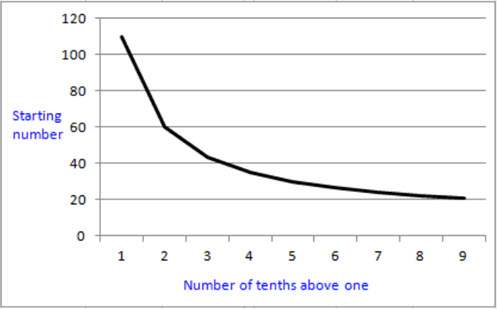Go to website

This lesson explores the connection between multiplying a number by a decimal and the addition of the same number and decimal multiplied by 10. By asking if this always happens, the students investigate place value using simple algebra. The resource includes the prompt, teacher notes and inquiry prompts.

Year level(s) Year 6, Year 7
Audience Teacher
Teaching strategies and pedagogical approaches Collaborative learning, Explicit teaching, Mathematics investigation, Questioning
Keywords decimal, place value, multiplication

## Curriculum alignment

Curriculum connections Critical and creative thinking, Numeracy
Strand and focus Algebra, Number, Build understanding
Topics Decimals, Place value, Multiples, factors and powers
AC: Mathematics (V9.0) content descriptions
AC9M6N06
Multiply and divide decimals by multiples of powers of 10 without a calculator, applying knowledge of place value and proficiency with multiplication facts; using estimation and rounding to check the reasonableness of answers

AC9M7A02
Formulate algebraic expressions using constants, variables, operations and brackets

Numeracy progression Number patterns and algebraic thinking (P6)
Multiplicative strategies (P9)
Number and place value (P9)